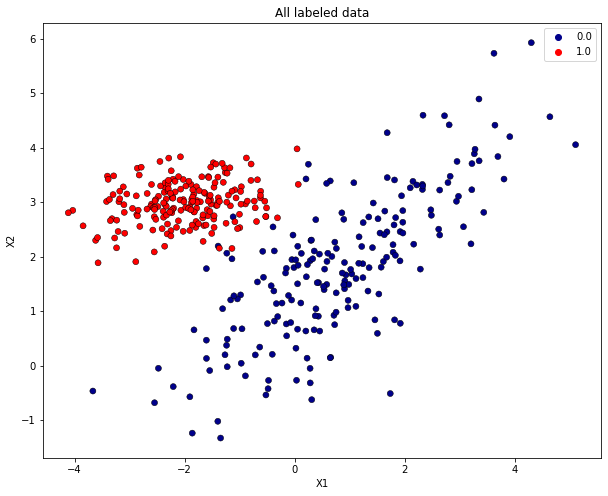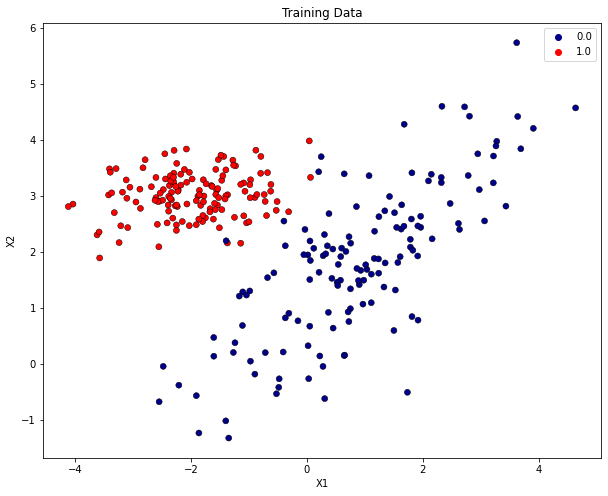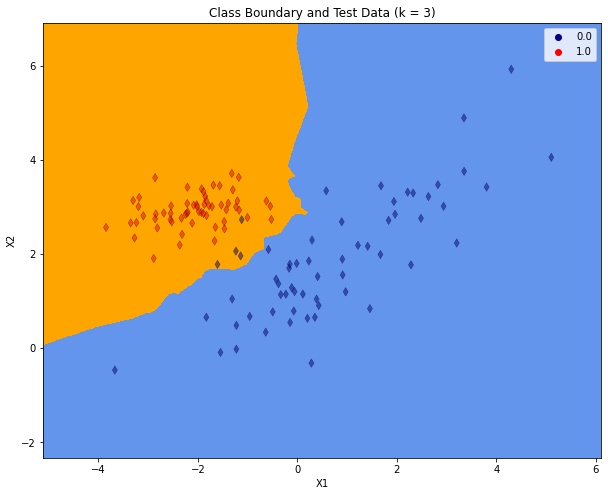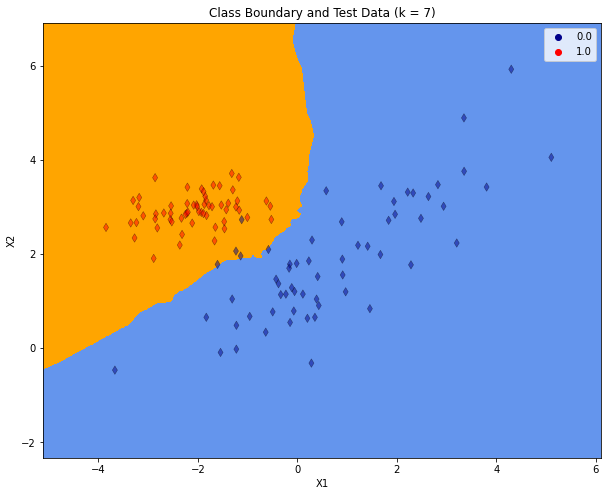# K - Nearest Neighbour Classification / Regression#

Probably the simplest supervised Machine Learning algorithm is K-Nearest Neighbour (K-NN). K-NN can be applied for both, classification and regression. Compared to other ML algorithms, K-NN is special because it is a non-parameteric ML algorithm, which actually has no training phase. It is therefore also called a Lazy Learner. All other ML algorithms, which will be introduced in this lecture, have a learning-phase during which either a class-specific data-distribution or a class-boundary is learned. After this learning phase the entire knowledge from the training-data set is encoded in some parameters, which uniquely define the class-specific distribution or the class-boundary. Not so with K-NN! In K-NN the entire set of labeled training data is stored. In the inference phase new data is classified by just comparing the new instance with all training-instances and deciding for the class, which most often appears in the set of k nearest training instances.

K-NN Classifier:

1. Training: Store the set of labeled training data $$T=\lbrace \mathbf{x}_t,r_t) \rbrace_{t=1}^N$$

2. Inference:

1. For a new input vector $$\mathbf{x}$$: Determine the set $$M(\mathbf{x},T,K)$$ of $$K$$ training instances from $$T$$, which are closest to $$\mathbf{x}$$.

2. Assign the class $$r$$ to $$\mathbf{x}$$, which most often appears in $$M(\mathbf{x},T,K)$$.

The number of neighbours $$K$$ is a hyperparameter. If $$K$$ is small (e.g. 1 or 3), the classifier is not robust w.r.t. outliers and possibly overfitted to training data. The higher the value of $$K$$, the smoother the class boundary and the better the generalisation. However, a too high value may yield underfitting.

Another important hyperparameter is the distance-function, e.g. Euclidean-, Manhattan- or any other Minkowski-distance.

K-NN Regression:

1. Training: Store the set of labeled training data $$T=\lbrace \mathbf{x}_t,r_t) \rbrace_{t=1}^N$$

2. Inference:

1. For a new input vector $$\mathbf{x}$$: Determine the set $$M(\mathbf{x},T,K)$$ of $$K$$ training instances from $$T$$, which are closest to $$\mathbf{x}$$.

2. Assign the value $$r$$ to $$\mathbf{x}$$ by interpolating over the values in $$M(\mathbf{x},T,K)$$.

For K-NN regression an additional hyperparameter is the interpolation method used to calculate the new value $$r$$. The simplest method is to just take the arithmetic mean over the values in the neighborhood.

K-NN Pros and Cons:

The drawbacks of K-NN are

• the large memory footprint, since all training data must be kept

• long inference time, since each new instance must be compared to all training instances in order to determine the nearest neighbours

For $$K=1$$ the bias is close to 0, but the variance is quite high. With increasing $$K$$ bias increases but variance decreases.

## K-NN Classification Demo#

In the reminder of this section the application of a K-NN classifier is demonstrated.

### Generate set of labeled training data#

In this demo we do not apply a real-world training data set. Instead we generate Gaussian-distributed 2-dimensional training vectors $$\mathbf{x}=(x_1,x_2)$$ for each of the 2 classes. The distributions of the 2 classes differ in their mean-vector and their covariance matrix. The code cells below implement the generation and visualisation of labeled data.

import numpy as np
from matplotlib import pyplot as plt
np.set_printoptions(precision=2)
from matplotlib.colors import ListedColormap
import seaborn as sns


Generate Gaussian-distributed 2-dimensional data for class 0:

np.random.seed(1234)

Numpoints=200
m1=1    #Mean of first dimension
m2=2    #Mean of second dimension
mean=np.array(([m1,m2]))
s11=1.7 #standard deviation of x1
s22=1.4 #standard deviation of x2
rho=0.8 #correlation coefficient between s11 and s22
#Determine covariance matrix from standard deviations
var11=s11**2
var22=s22**2
var12=rho*s11*s22
var21=var12
cov=np.array(([var11,var12],[var21,var22]))
print("Configured mean: ")
print(mean)
print("Configured covariance: ")
print(cov)
pointset0=np.random.multivariate_normal(mean,cov,Numpoints,)
print("First samples of pointset:")
print(pointset0[:20,:])

Configured mean:
[1 2]
Configured covariance:
[[2.89 1.9 ]
[1.9  1.96]]
First samples of pointset:
[[ 0.73  0.75]
[-1.23 -0.02]
[ 1.81  3.41]
[-0.15  0.55]
[ 1.92  0.78]
[-1.31  1.04]
[ 0.28 -0.32]
[ 1.55  2.43]
[ 0.21  1.63]
[-0.52 -0.54]
[ 1.61  1.91]
[ 0.45  2.05]
[-0.97  0.04]
[ 0.65  0.15]
[ 0.86  2.8 ]
[ 1.51  2.7 ]
[-1.17  1.21]
[-0.37  0.82]
[ 0.93  1.67]
[-1.39  2.19]]


Generate Gaussian-distributed 2-dimensional data for class 1:

Numpoints=200
m1=-2    #Mean of first dimension
m2=3   #Mean of second dimension
mean=np.array(([m1,m2]))
s11=0.8 #standard deviation of x1
s22=0.4 #standard deviation of x2
rho=0.1 #correlation coefficient between s11 and s22
#Determine covariance matrix from standard deviations
var11=s11**2
var22=s22**2
var12=rho*s11*s22
var21=var12
cov=np.array(([var11,var12],[var21,var22]))
print("Configured mean: ")
print(mean)
print("Configured covariance: ")
print(cov)
pointset1=np.random.multivariate_normal(mean,cov,Numpoints)
print("First samples of pointset:")
print(pointset1[:20,:])

Configured mean:
[-2  3]
Configured covariance:
[[0.64 0.03]
[0.03 0.16]]
First samples of pointset:
[[-1.79  2.65]
[-2.25  2.48]
[-1.83  2.83]
[-2.83  3.5 ]
[-2.88  3.12]
[-1.26  3.54]
[-2.39  2.72]
[-1.43  2.94]
[-2.48  3.11]
[-0.52  2.74]
[-1.4   3.  ]
[-2.23  3.08]
[-1.78  2.89]
[-2.36  2.95]
[-1.63  3.16]
[-1.17  2.93]
[-3.09  2.43]
[-0.79  3.7 ]
[-1.52  2.96]
[-3.32  2.7 ]]


Assign class labels and vertically stack class 0 and class 1 data. The array of all input vectors is $$X$$ and the vector of all labels is $$y$$. The class-index of the $$i.th$$ row in $$X$$ is the value of the $$i.th$$ component in $$y$$:

X=np.vstack([pointset0,pointset1])
y=np.transpose(np.hstack([np.zeros(Numpoints),np.ones(Numpoints)]))


Visualize all labeled data. Data of class 0 has blue, data of class 1 red markers.

h = .02  # step size in the mesh
# Create color maps
cmap_light = ListedColormap(['cornflowerblue','orange'])
cmap_bold = ['darkblue','red']

plt.figure(figsize=(10, 8))
sns.scatterplot(x=X[:, 0], y=X[:, 1],hue=y,palette=cmap_bold, alpha=1.0, edgecolor="black")
plt.title("All labeled data")
plt.xlabel("X1")
plt.ylabel("X2")
plt.show()### Split labeled data in training- and test-partition#

The scikit-learn function train_test_split() shuffles the set of all labeled data and returns two partions of input vectors, X_train and X_test and the corresponding class-labels y_train and y_test.

from sklearn.model_selection import train_test_split
from sklearn import neighbors

X.shape

(400, 2)

y.shape

(400,)

X_train, X_test, y_train, y_test = train_test_split(X, y, test_size=0.3, random_state=412)


Visualize training data: The plot below contains only those input-vectors from $$X$$, which has been assigned to the training-partition $$X$$.

plt.figure(figsize=(10, 8))
sns.scatterplot(x=X_train[:, 0], y=X_train[:, 1],hue=y_train,palette=cmap_bold, alpha=1.0, edgecolor="black")
plt.title("Training Data")
plt.xlabel("X1")
plt.ylabel("X2")
plt.show()### Train the K-NN classifier#

All machine learning algorithms implemented in scikit-learn provide a .fit(X_train, y_train)-method, in which the training phase, i.e. the fitting of a model to the given training-data, is implemented. As already described above, in the case of the K-NN algorithm, there is no training-phase. Here, fit() just saves the given training data accordingly. The model is the set of training data itself. In this example the chosen number of neighbors is $$K=3$$.

K=3
clf = neighbors.KNeighborsClassifier(K)
clf.fit(X_train, y_train)

KNeighborsClassifier(n_neighbors=3)


After training the resulting class boundary is plotted. The plot also contains the test-data. For plotting the decision boundary a class-specific color will be assigned to each point in the mesh $$[x_{min}, x_{max}] \times [y_{min}, y_{max}]$$.

x_min, x_max = X[:, 0].min() - 1, X[:, 0].max() + 1
y_min, y_max = X[:, 1].min() - 1, X[:, 1].max() + 1
xx, yy = np.meshgrid(np.arange(x_min, x_max, h),
np.arange(y_min, y_max, h))
Z = clf.predict(np.c_[xx.ravel(), yy.ravel()])

# Put the result into a color plot
Z = Z.reshape(xx.shape)
plt.figure(figsize=(10, 8))
plt.contourf(xx, yy, Z, cmap=cmap_light)

# Plot also the test points
sns.scatterplot(x=X_test[:, 0], y=X_test[:, 1],hue=y_test,palette=cmap_bold,marker="d",alpha=0.5,edgecolor="black")
plt.xlim(xx.min(), xx.max())
plt.ylim(yy.min(), yy.max())
plt.title("Class Boundary and Test Data (k = %i)"% (K))
plt.xlabel("X1")
plt.ylabel("X2")
plt.show()### Evaluate the classifier on Test-data#

y_pred=clf.predict(X_test)

from sklearn.metrics import accuracy_score,confusion_matrix

accuracy_score(y_test,y_pred)

0.9666666666666667

confusion_matrix(y_test,y_pred)

array([[56,  4],
[ 0, 60]])


As can be seen in the confusion matrix, all class-1 data has been classified correctly. But there are 4 out of 60 class-0 instances, which are assigned to the wrong class. In the visualisation above, these errors are the blue markers in the orange region.

### Repeat Training and Evaluation for $$K=9$$ neighbors#

K=7
clf = neighbors.KNeighborsClassifier(K)
clf.fit(X_train, y_train)

KNeighborsClassifier(n_neighbors=7)

x_min, x_max = X[:, 0].min() - 1, X[:, 0].max() + 1
y_min, y_max = X[:, 1].min() - 1, X[:, 1].max() + 1
xx, yy = np.meshgrid(np.arange(x_min, x_max, h),
np.arange(y_min, y_max, h))
Z = clf.predict(np.c_[xx.ravel(), yy.ravel()])

# Put the result into a color plot
Z = Z.reshape(xx.shape)
plt.figure(figsize=(10, 8))
plt.contourf(xx, yy, Z, cmap=cmap_light)

# Plot also the test points
sns.scatterplot(x=X_test[:, 0], y=X_test[:, 1],hue=y_test,palette=cmap_bold,marker="d",alpha=0.5,edgecolor="black")
plt.xlim(xx.min(), xx.max())
plt.ylim(yy.min(), yy.max())
plt.title("Class Boundary and Test Data (k = %i)"% (K))
plt.xlabel("X1")
plt.ylabel("X2")
plt.show()y_pred=clf.predict(X_test)

accuracy_score(y_test,y_pred)

0.9666666666666667

confusion_matrix(y_test,y_pred)

array([[56,  4],
[ 0, 60]])


As the plot above shows, for the increased $$K$$ the class-boundary becomes smoother. But there are still 4 misclassified instances in the test-partition.